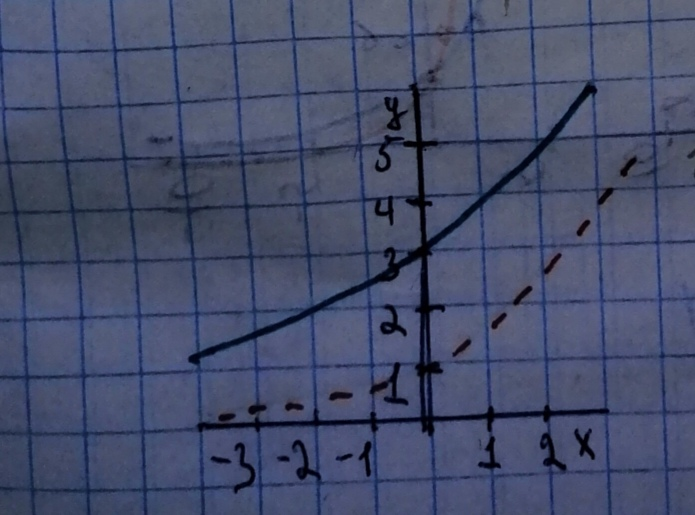# Begin by graphing f(x)=2^{x}. Then use transformations of this graph to graph the given function. Be sure to graph and give equations of the asymptotes. Use the graphs to determine each function's domain and range. If applicable, use a graphing utility to confirm your hand-drawn graphs. g(x)=2^{x}=2^{x} + 2Question
Transformations of functionsBegin by graphing
$$\displaystyle{f{{\left({x}\right)}}}={2}^{{{x}}}.$$
Then use transformations of this graph to graph the given function. Be sure to graph and give equations of the asymptotes. Use the graphs to determine each function's domain and range. If applicable, use a graphing utility to confirm your hand-drawn graphs.
$$\displaystyle{g{{\left({x}\right)}}}={2}^{{{x}}}={2}^{{{x}}}\ +\ {2}$$2021-02-15
Step 1Step 2
Graph of $$\displaystyle{2}^{{{x}}}$$ is shown as a dotted curve.
The graph of $$\displaystyle{2}^{{{x}}}\ +\ {2}$$ is the graph of $$\displaystyle{2}^{{{x}}}$$ translated left by two units.
Its asymplote has equation $$\displaystyle{y}={2}$$

### Relevant QuestionsBegin by graphing
$$\displaystyle{f{{\left({x}\right)}}}={2}^{{{x}}}.$$
Then use transformations of this graph to graph the given function. Be sure to graph and give equations of the asymptotes. Use the graphs to determine each function's domain and range. If applicable, use a graphing utility to confirm your hand-drawn graphs.
$$\displaystyle{g{{\left({x}\right)}}}={2}^{{{x}\ +\ {2}}}$$Begin by graphing
$$\displaystyle{f{{\left({x}\right)}}}={2}^{{{x}}}.$$
Then use transformations of this graph to graph the given function. Be sure to graph and give equations of the asymptotes. Use the graphs to determine each function's domain and range. If applicable, use a graphing utility to confirm your hand-drawn graphs.
$$\displaystyle{g{{\left({x}\right)}}}={\frac{{{1}}}{{{2}}}}\ \cdot\ {2}^{{{x}}}$$Begin by graphing
$$\displaystyle{f{{\left({x}\right)}}}={2}^{{{x}}}$$
Then use transformations of this graph to graph the given function. Be sure to graph and give equations of the asymptotes. Use the graphs to determine each function's domain and range. If applicable, use a graphing utility to confirm your hand-drawn graphs.
$$\displaystyle{g{{\left({x}\right)}}}=-{2}^{{{x}}}$$Begin by graphing
$$\displaystyle{f{{\left({x}\right)}}}={2}^{{{2}}}$$
Then use transformations of this graph to graph the given function. Be sure to graph and give equations of the asymptotes. Use the graphs to determine each function's domain and range. If applicable, use a graphing utility to confirm your hand-drawn graphs.
$$\displaystyle{g{{\left({x}\right)}}}={2}^{{-{x}}}$$Begin by graphing $$\displaystyle{f{{\left({x}\right)}}}=\ {{\log}_{{{2}}}{x}}$$ Then use transformations of this graph to graph the given function. What is the vertical asymptote? Use the graphs to determine each function's domain and range. $$\displaystyle{g{{\left({x}\right)}}}={\frac{{{1}}}{{{2}}}}\ {{\log}_{{{2}}}{x}}$$Begin by graphing $$\displaystyle{f{{\left({x}\right)}}}=\ {{\log}_{{{2}}}{x}}$$ Then use transformations of this graph to graph the given function. What is the vertical asymptote? Use the graphs to determine each function's domain and range. $$\displaystyle{g{{\left({x}\right)}}}=\ -{2}\ {{\log}_{{{2}}}{x}}$$Begin by graphing
$$\displaystyle{f{{\left({x}\right)}}}={{\log}_{{{2}}}{x}}$$
Then use transformations of this graph to graph the given function. What is the vertical asymptote? Use the graphs to determine each function s domain and range.
$$\displaystyle{g{{\left({x}\right)}}}={\frac{{{1}}}{{{2}}}}{{\log}_{{{2}}}{x}}$$Begin by graphing $$\displaystyle{f{{\left({x}\right)}}}={{\log}_{{{2}}}{x}}$$ Then use transformations of this graph to graph the given function. What is the vertical asymptote? Use the graphs to determine each functions domain and range. $$\displaystyle{g{{\left({x}\right)}}}=\ -{2}{{\log}_{{{2}}}{x}}$$Begin by graphing $$\displaystyle{f{{\left({x}\right)}}}=\ {{\log}_{{{2}}}{x}}$$ Then use transformations of this graph to graph the given function. What is the graph's x-intercept? What is the vertical asymptote? Use the graphs to determine each function's domain and range. $$\displaystyle{g{{\left({x}\right)}}}=\ {{\log}_{{{2}}}{\left({x}\ -\ {2}\right)}}$$Graph f and g in the same rectangular coordinate system. Use transformations of the graph f of to obtain the graph of g. Graph and give equations of all asymptotes. Use the graphs to determine each function's domain and range. $$\displaystyle{f{{\left({x}\right)}}}={2}^{{{x}}}\text{and}{g{{\left({x}\right)}}}={2}^{{{x}-{1}}}$$